# Algebra - math word problems

#### Number of problems found: 2655

• Circle arcCircle segment has a circumference of 135.26 dm and 2096.58 dm2 area. Calculate the radius of the circle and size of central angle.
• Geometric progression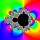Fill 4 numbers between 4 and -12500 to form geometric progression.
• Two doctorsDoctor A will determine the correct diagnosis with a probability 93% and doctor B with a probability 79%. Calculate probability of correct diagnosis if patient is diagnosed by both doctors.
• GardenTrapezoid garden has parallel sides 19 m and 24 m. Its area is 193.5 square meters. What is the width of the garden?
• Computer revolution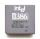When we started playing with computers, the first processor, which I remember was the Intel 8080 from 1974, with the performance of 0.5 MIPS. Calculate how much percent a year rose CPU performance when Intel 486DX from 1992 has 54 MIPS. What wou
• Logarithmic equation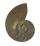Solve equation: log33(3x + 21) = 0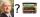Ladislav was reward and went to conference in Amsterdam. Conferense fee was € 3484. Calculate how many books Ladislav could buy at the price of 48 and 52 euros if he don't want fill whole home library and he can buy only 70 books?
• ShapePlane shape has a maximum area 677 mm2. Calculate its perimeter if perimeter is the smallest possible.
• Number ratio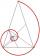Calculate two positive numbers that its ratio is 6:6 and difference was 0.
• Angles in triangleThe triangle is ratio of the angles β:γ = 6:8. Angle α is 40° greater than β. What are the size of angles of the triangle?
• PlaygroundRectangular playground is fenced with 38 m long netting. Its width is 7 m. Calculate its length.
• Rectangle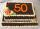Area of rectangle is 3002. Its length is 41 larger than the width. What are the dimensions of the rectangle?
• Square diagonalCalculate the length of the square diagonal if the perimeter is 476 cm.
• Salary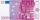Lawyer got to pay 840 Euros in banknotes of 20 and 50 Eur. Total got 18 banknotes. How many was which?
• Chickens and rabbitsIn the yard were chickens and rabbits. Together they had 40 heads and 106 legs. How many chickens and how many rabbits was in the yard?
• TrianglesEquilateral triangle with side 40 cm has the same perimeter as an isosceles triangle with arm of 45 cm. Calculate the base x of an isosceles triangle.
• TrapezoidCalculate area of trapezoid ABCD with sides |AD|= 68 cm, |DC|=35 cm, |CB|=12 cm, |AB|=35 cm..Solve quadratic equation: 2x2-58x+396=0Find two numbers whose difference and ratio is 2.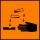The barrel is 199 litres of 38% acid. How many percent acid occurs when 61 litres revoke and replace it with the same amount of water?